# College Algebra : Review and Other Topics

## Example Questions

← Previous 1 3 4 5 6 7 8 9 13 14

### Example Question #1 : Simplifying Exponents

Simplify the expression: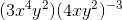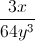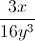Cannot be simplified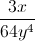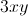Explanation:

Begin by distributing the exponent through the parentheses. The power rule dictates that an exponent raised to another exponent means that the two exponents are multiplied: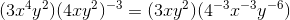Any negative exponents can be converted to positive exponents in the denominator of a fraction: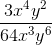The like terms can be simplified by subtracting the power of the denominator from the power of the numerator: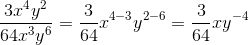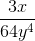### Example Question #1 : Generate Equivalent Numerical Expressions: Ccss.Math.Content.8.Ee.A.1

Simplify: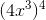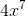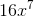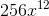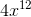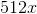Explanation:

Use the power rule to distribute the exponent: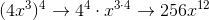### Example Question #1 : Integer Exponents

Simplify the following expression: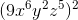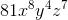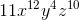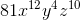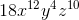Explanation:

Simplify the following expression:To raise exponents to another power, we need to multiply them: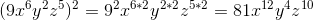So we get:### Example Question #1 : Generate Equivalent Numerical Expressions: Ccss.Math.Content.8.Ee.A.1

Simplify.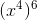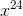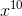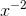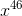Explanation:

When an exponent is being raised by another exponent, we just multiply the powers of the exponents and keep the base the same.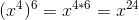### Example Question #3 : Integer Exponents

Simplify.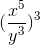Explanation:

When an exponent is being raised by another exponent, we just multiply the powers of the exponents and keep the base the same.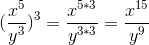### Example Question #4 : Integer Exponents

Combine the following terms: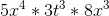Explanation:

Combine the following terms:We need to multiply three terms together. We should recognize that the t must be left alone, because it cannot be combined with the x's.

First, multiply all three coefficients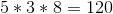Next, combine the x's. Because we are multiplying, we will add the exponents.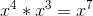Now, put it all together to  get: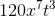### Example Question #5 : Integer Exponents

Evaluate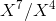Explanation:

When common variables with exponents are divided, the exponents are subtracted.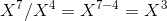### Example Question #6 : Integer Exponents

Evaluate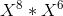Explanation:

When like terms with exponents are multiplied, you add the exponents.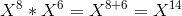### Example Question #7 : Integer Exponents

Evaluate the following: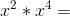Explanation:

When similar terms raised to n are multiplied, simply add the exponents. Thus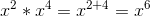### Example Question #8 : Integer Exponents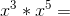Explanation:

When similar terms raised to n are multiplied, simply add the exponents. Thus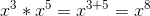← Previous 1 3 4 5 6 7 8 9 13 14

### All College Algebra Resources